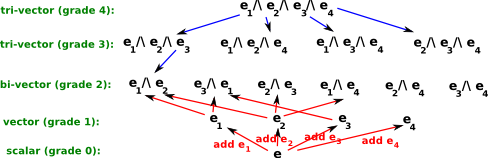# Maths - 4D Clifford / Geometric Algebra

## Prerequisites

If you are not familiar with vectors this subject you may like to look at the following page first:

## Description

For 4 dimensions can be generated by 4 basis vectors, e1, e2, e3 and e4 this means that a 4D multivector is represented by 16 scalar numbers:The pages below this explain in detail:

 Choice of bivector and tri-vector basis Here I have described how the order of the indexes was chosen. For instance, why don't we use e214 instead of e124? Unlike 3D multivectors there is no correspondence with cross multiplication as cross multiplication does not apply in 4D. Also I cant work out how to make the multiplication table symmetrical. Also its not possible to make all the e1234 terms on the bottom left to top right diagonal positive as some of these terms anti-commute. Transform Arithmetic This gives the rules for addition, subtraction and multiplication of 4D multivectors. Functions This shows the dual, reverse and conjugate functions of of 4D multivectors. isometries code pauli An alternative notation using a matrix to represent each multvector

The following table explains the notation for each of the 16 elements as follows:

full
shortened
0=unit scalar 1 e
1=unit length base vectors e1 e1
e2 e2
e3 e3
e4 e4
2=unit length base bivectors e1^ e2 e12 e12
e1^ e3 e13 e13
e1^ e4 e14 e14
e2^ e3 e23 e23
e4^ e2 e42 e42
e3^ e4 e34 e34
3=unit length base tri-vector e3^ e2^ e1 e321 e321
e1^ e2^ e4 e124 e124
e4^ e3^ e1 e431 e431
e2^ e3^ e4 e234 e234
4=unit length base tri-vector e1^ e2^ e3^ e4 e1234 e1234

So in this case the number of dimensions is:

• scalar dimensions=1
• vector dimensions=4
• bivector dimensions=6
• tri-vector dimensions=4
 metadata block see also: Correspondence about this page Book Shop - Further reading. Where I can, I have put links to Amazon for books that are relevant to the subject, click on the appropriate country flag to get more details of the book or to buy it from them.Geometric Computing for Perception Action Systems: Concepts, Algorithms, and Scientific Applications (Hardcover). This is the only book I have so far come across that has a reasonable explanation of 'motors' and why it is useful to use 4D Geometric algebra to represent kinematics of solid bodies (in chapter 2). The book is quite a slim volume considering that it covers both fundamental concepts and practical applications. Therefore I think you will need to have a good understanding of Geometric Algebra before starting on this book. Specific to this page here: